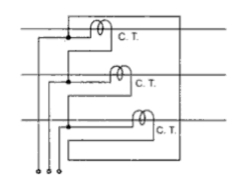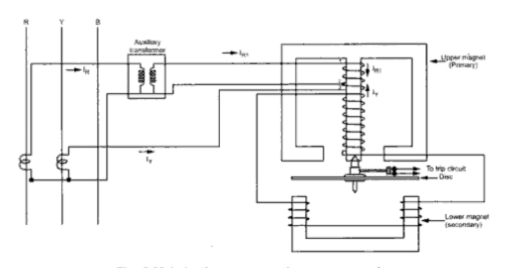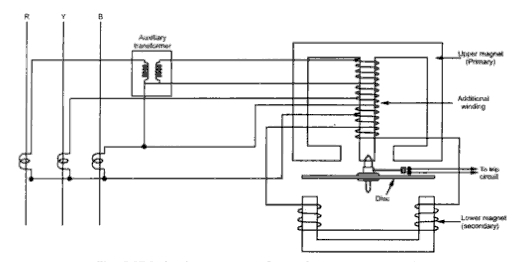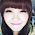### Negative Sequence Relays

Generator Protection Part 12
1. Negative Sequence Relays
The negative relays are also called phase unbalance relays because these relays provide protection against negative sequence component of unbalanced currents existing due to unbalanced loads or phase-phase faults. The unbalanced currents are dangerous from generators and motors point of view as these currents can cause overheating. Negative sequence relays are generally used to give protection to generators and motors against unbalanced currents.
A negative sequence relay has a filter circuit which is operative only for negative sequence components. Low order of over current also can cause dangerous situations hence a negative sequence relay has low current settings. The earth relay provides protection for phase to earth fault but not for phase to phase fault. A negative sequence relay provides protection against phase to phase faults which are responsible to produce negative sequence components.
The Fig. 1 shows the schematic arrangement of negative phase sequence relay.Fig. 1 Negative phase sequence relay
Basically it consists of a resistance bridge network. The magnitudes of the impedances of all the branches of the network are equal. The impedances Z1 and Z3 are purely resistive while the impedances Z2 and Z4 are the combinations of resistance and reactance. The currents in the branches Z2 and Z4 lag by 60o from the currents in the branches Z1 and Z3. The vertical branch B-D consists of inverse time characteristics relay. The relay has negligible impedance.Fig. 2
The current IR gets divided into two equal parts I1 and I2. And I2 lags I1 by 60o. The phasor diagram is shown in the Fig. 2.
Ī1 + Ī2=  Īrs
Let                       I1 = I2 = I
The perpendicular is drawn from point A on the diagonal meeting it at point B, as shown in the Fig. 2. This bisects the diagonal.
...                        OB = IR /2
Now in triangle OAB,
cos 30 = OB/OA
...                        √3/2 = (IR/2)/I
...                         I = IR/√3 = I1 = I2                                          ............(1)
Now I1 leads IR by 30o while I2 lags IR by 30o.
Similarly the current IB gets divided into two equal parts I3 and I4. The current I3 lags I4 by 60o. From equation (1) we can write,
IB /√3 = I3 = I4                                                    ...............(2)
The current I4 leads by IB while current I3 lags IB by 30o.
The current entering the relay at the junction point B in the Fig. 1 is the vector sum of , and .
Irelay  = Ī1 + Ī3 + ĪY
= IY + (IR/√3) (leads IR by 30o) + IB/√3(lags IB by 30o)
The vector sum is shown in the Fig. 3 when the load is balanced and no negative sequence currents exist.Fig. 3
It can be seen from the Fig. 3 that,
Ī1 + Ī3 = -ĪY
...                               Ī1 + Ī3 + ĪY = 0
Thus the current entering the relay at point B is zero. Similarly the resultant current at junction D is also zero. Thus the relay is inoperative for a balanced system.
Now consider that there is unbalanced load on generator or motor due to which negative sequence currents exist. The phase sequence of C.T. secondary currents is as shown in the Fig. 4(a). The vector diagram of I1, I3 and IY is shown in the Fig. 4(b) under this condition.
The component I1 and I3 are equal and opposite to each other at the junction point B. Hence I1 and I3 cancel each other. Now the relay coil carries the current IY and when this current is more than a predetermined value, the relay trips closing the contacts of trip circuit which opens the circuit breaker.Fig. 4   Negative sequence current

Zero Sequence Currents : The zero sequence components of secondary currents are shown in the Fig. 5(a). We know that,Fig. 5  Zero sequence currents
ĪR = Ī1 + Ī2
ĪB = Ī3 + Ī4
These sums are shown in the Fig. 5(b) and (c). It can be seen from the Fig. 5(d) that,
Ī1 + Ī3 = ĪY in phase with IY
The total current through relay is Ī1 + Ī3Y. Thus under zero sequence currents the total current of twice the zero sequence current flows through the relay. Hence the relay operates to open the circuit breaker.
To make the relay sensitive to only negative sequence currents by making it inoperative under the influence of zero sequence currents is possible by connecting the current transformers in delta as shown in the Fig. 6. Under delta connection of current transformers, no zero sequence current can flow in the network.Fig. 6   Delta connection of C.T.s

1.1 Induction Type Negative Sequence Relay
Another commonly used negative sequence relay is induction type. Its construction is similar to that of induction type over current relay. The schematic diagram of this type of relay is shown in the Fig. 7.Fig. 7 Induction type negative sequence relay

The central limb of upper magnet carries the primary which has a centre tap. Due to this, the primary winding has three terminal 1, 2 and 3. The section 1-2 is energized from the secondary of an auxiliary transformer to R-phase. The section 2-3 is directly energized from the Y-phase current.
The auxiliary transformer is a special device having an air gap in its magnetic circuit. With the help of this, the phase angle between its primary and secondary can be easily adjusted. In practice it is adjusted such that output current lags by 120o rather than usual 180ofrom the input.
So,                   Ix = Input current of auxiliary transformer
IR1 = Output current of auxiliary transformer
and                   IR1 lags I by 120o
Hence the relay primary carries the current which is phase difference of IR1 and IR .
Positive Sequence Current : The C.T. secondary currents are shown in the Fig. 8(a). The Fig. 8(b) shows the position of vector IR1 lagging I by120o. The Fig. 8(c) shows the vector sum of IR1 and - IY.
The phase difference of IR1 and IY is the vector sum of IR1 and - IY. It can seen from the Fig. 8(c) that the resultant is zero. Thus the relay primary current is zero and relay is inoperative for positive sequence currents.Fig. 8 Positive sequence currents

Negative Sequence Currents : The C.T. secondary currents are shown in the Fig.. 9(a). The Fig. 9(b) shows the position of IR1 lagging I by 120o. The Fig. 9(c) shows the vector difference of IR1 and IY which is the relay current.
Under negative sequence currents, the vector difference of IR1 and IY results into a current I as shown in the Fig. 9(c). This current flows through the primary coil of the relay.Fig. 9  Negative sequence currents

Under the influence of current I, the relay operates. The disc rotates to close the trip contacts and opens the circuit breaker.
This relay is inoperative for zero sequence currents. But the relay can be made operative for the flow of zero sequence currents also by providing an additional winding on the central limb of the upper magnet of the relay. This winding is connected in the residual circuit of three line C.T. This relay is called induction type negative and zero sequence relay.
The schematic arrangement of induction type negative and zero sequence relay is shown in the Fig.10.Fig. 10 Induction type negative and zero sequence relay

Sponsored links :

#### 6 comments:

1.Once you replace negative thoughts with positive ones, you'll start having positive results. See the link below for more info.

#negative
www.matreyastudios.com

2.Really appreciate your work guys,Nice explanation with proper diagrams.Thank you!!

3.This is very impressive information to me starting job for relay. Thank you!!

4.Practical circuit is not working :( (Fig. 1)

5.I just wanna say thank you for sharing the content and wish you all the best for your website and your whole team.

Count
www.imarksweb.org

6.